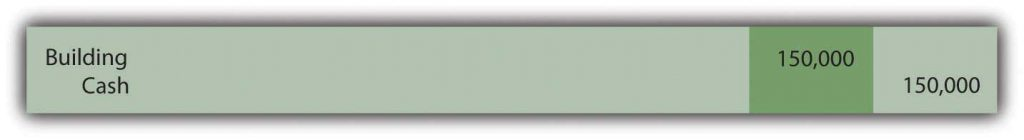# 4.5: Recording Expenditures That Affect Older Assets

$$\newcommand{\vecs}{\overset { \rightharpoonup} {\mathbf{#1}} }$$ $$\newcommand{\vecd}{\overset{-\!-\!\rightharpoonup}{\vphantom{a}\smash {#1}}}$$$$\newcommand{\id}{\mathrm{id}}$$ $$\newcommand{\Span}{\mathrm{span}}$$ $$\newcommand{\kernel}{\mathrm{null}\,}$$ $$\newcommand{\range}{\mathrm{range}\,}$$ $$\newcommand{\RealPart}{\mathrm{Re}}$$ $$\newcommand{\ImaginaryPart}{\mathrm{Im}}$$ $$\newcommand{\Argument}{\mathrm{Arg}}$$ $$\newcommand{\norm}{\| #1 \|}$$ $$\newcommand{\inner}{\langle #1, #2 \rangle}$$ $$\newcommand{\Span}{\mathrm{span}}$$ $$\newcommand{\id}{\mathrm{id}}$$ $$\newcommand{\Span}{\mathrm{span}}$$ $$\newcommand{\kernel}{\mathrm{null}\,}$$ $$\newcommand{\range}{\mathrm{range}\,}$$ $$\newcommand{\RealPart}{\mathrm{Re}}$$ $$\newcommand{\ImaginaryPart}{\mathrm{Im}}$$ $$\newcommand{\Argument}{\mathrm{Arg}}$$ $$\newcommand{\norm}{\| #1 \|}$$ $$\newcommand{\inner}{\langle #1, #2 \rangle}$$ $$\newcommand{\Span}{\mathrm{span}}$$$$\newcommand{\AA}{\unicode[.8,0]{x212B}}$$

### Learning Objectives

At the end of this section, students should be able to meet the following objectives:

1. Know when expenditures must be capitalized for an asset that has been in use for some time and the impact on future depreciation expense calculations.

Question: Assume that a building is acquired at a cost of $1,257,500. Assume further that it has an expected life of twenty years and straight-line depreciation is applied with no residual value. Thus, after eight years, accumulated depreciation is$503,000 ($1,257,500 × 8 years/20 years). At that point, the company spends an additional$150,000 on the building. Should an expenditure associated with property and equipment that is already in use be capitalized (added to the asset account) or expensed immediately?

Answer: The answer to this question depends on the impact that this work has on the building. In many cases, additional money is spent simply to keep the asset operating with no change in expected life or improvement in future productivity. Such costs are recorded as maintenance expense if they were anticipated or repair expense if unexpected. For example, changing the oil in a truck at regular intervals is a maintenance expense whereas fixing a dent from an accident is a repair expense. This distinction has no impact on reported income.

Figure 4.7 Recording of Cost to Maintain or Repair AssetHowever, if the $150,000 cost increases the future operating capacity of the asset, the amount should be capitalized. The building might have been made bigger, more efficient, more productive, or less expensive to operate. If the asset has actually been improved by the cost incurred, historical cost is raised. Figure 4.8 Cost Capitalized Because of Increase in Operating CapacityAssuming that no change in either the useful life or the residual value occurs as a result of this work, depreciation expense will be$75,375 in each of the subsequent twelve years. The newly increased book value is simply allocated over the useful life that remains.

($1,257,500 +$150,000 – $503,000)/12 remaining years =$75,375  (New book value divided by remaining useful life)

Another possibility does exist. The $150,000 might extend the building’s life without creating any other improvement. Because the building will now generate revenue for a longer period of time than previously expected, this cost is capitalized. A clear benefit has been gained from the amount spent. The asset is not physically bigger or improved but its estimated life has been extended. Consequently, the building is not increased directly, but instead, accumulated depreciation is reduced. In effect, this expenditure has recaptured some of the previously expensed utility. Figure 4.9 Cost Capitalized Because Expected Life Is ExtendedAssuming the$150,000 payment extends the remaining useful life of the building from twelve to eighteen years with no accompanying change in residual value, depreciation expense will be $50,250 in each of these remaining eighteen years. Once again, the book value has increased but, in this situation, the life of the asset has also been lengthened. reduced accumulated depreciation:$503,000 – $150,000 =$353,000
adjusted net book value: $1,257,500 –$353,000 = $904,500 annual depreciation:$904,500/18 years = $50,250 Still the new book value divided by remaining useful life ### Check Yourself Vlad, Inc. owns an asset that originally cost$80,000 and they have accumulated $55,000 in depreciation over the years. Vlad spends$26,000 in cash on making the asset more efficient and better at the beginning of 2020.  This improvement will make it so the asset will have a remaining useful life of 5 years with no residual value.  What depreciation amount should Vlad record for this asset in 2020 using the straight line method?

A.  $10,200 B.$26,000

C. $35,000 D.$5,100

The answer is A.  With the improvement making the asset last longer and more efficient the $26,000 will be added to the original cost. The new book value will be 80,000 + 26,000 – 55,000 =$51,000.  This will be divided by the remaining useful life of 5 years to get \$10,200.

### Key Takeaways

Subsequent costs incurred in connection with property and equipment are capitalized if the asset has been made bigger or better in some way. If the length of the remaining useful life is extended, capitalization is established by reducing accumulated depreciation.

4.5: Recording Expenditures That Affect Older Assets is shared under a not declared license and was authored, remixed, and/or curated by LibreTexts.